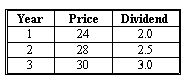### CFA Practice Question

There are 96 practice questions for this study session.

### CFA Practice Question

An investor plans to invest in a stock, but has not decided yet whether his investment will have one-, two-, or three-period horizon. The company's stock is currently trading at \$27.5 and current dividend is \$3. His forecast of dividend and stock price (at the end of each year) is given as below:Provided that the required rate of return on the stock is 10%, which investment horizons will make its stock attractive for the investor?
A. Three year
B. Two year
C. One year
Explanation: One-period DDM: V0 = D1 / (1 + r) + P1 / (1 + r) = 2 / (1 + 0.1) + 24 / (1 + 0.1) = \$23.6.

Two-period DDM: V0 = D1 / (1 + r) + D2 / (1 + r)2 + P2 / (1 + r)2 = 2 / (1 + 0.1) + 2.5 / (1 + 0.1)2 + 28 / (1 + 0.1)2 = \$27.0.

Three-period DDM: V0 = D1 / (1 + r) + D2 / (1 + r)2 + D3 / (1 + r)3 + P3 / (1 + r)3 = 2 / (1 + 0.1) + 2.5 / (1 + 0.1)2 + 3.0 / (1 + 0.1)3 + 30 / (1 + 0.1)3= \$28.7.

Depending on the investor's investment horizon, the stock value will be \$23.6, \$27.0, or \$28.7. Since the stock is currently trading at \$27.5, the stock is attractive only in the three-year period. Although the company will trade higher than its current price in two years' time, the price appreciation is not enough to compensate the investor for the time value of money.